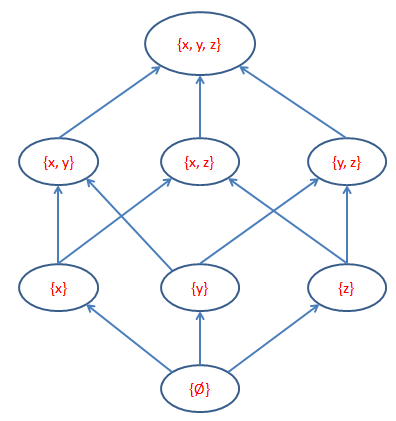# Number Of Subsets (Powersets) Calculator

Calculator will compute the subsets for given set.

 Enter Set Value (separate by comma,)

A set is called the power set of any set, if it contains all subsets of that set.

P(S) is the notation for representing any power set of the set.

### for example:

for the set S={1,2,3,4,5} means that S has 5

P(S) = 2n = 25 = 32